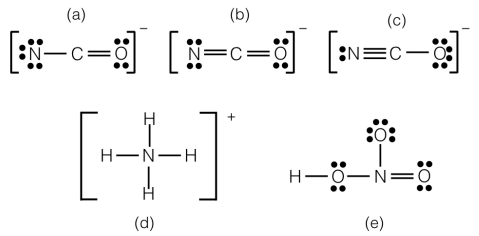# Problem: In which of the Lewis structures below does the nitrogen atom have a formal charge of −2?

###### FREE Expert Solution
100% (108 ratings)
###### FREE Expert Solution

We’re being asked to determine the structure where nitrogen has a formal charge of –2

For this problem, we need to compare the formal charge of nitrogen in each structure.

Recall that the formula for the formal charge is:

100% (108 ratings)###### Problem Details

In which of the Lewis structures below does the nitrogen atom have a formal charge of −2?What scientific concept do you need to know in order to solve this problem?

Our tutors have indicated that to solve this problem you will need to apply the Formal Charge concept. You can view video lessons to learn Formal Charge. Or if you need more Formal Charge practice, you can also practice Formal Charge practice problems.

What is the difficulty of this problem?

Our tutors rated the difficulty ofIn which of the Lewis structures below does the nitrogen ato...as low difficulty.

How long does this problem take to solve?

Our expert Chemistry tutor, Jules took 1 minute and 40 seconds to solve this problem. You can follow their steps in the video explanation above.

What professor is this problem relevant for?

Based on our data, we think this problem is relevant for Professor Beshir's class at UCONN.